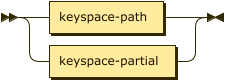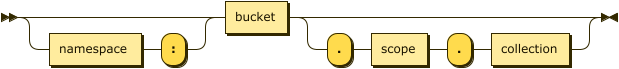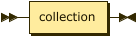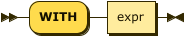# Update Statistics for Index Expressions

+
You can use the `UPDATE STATISTICS` statement to gather statistics for an index key expression.

## Purpose

The `UPDATE STATISTICS` statement provides a syntax which enables you to gather statistics for an index key expression.

When you use an index with a query, you typically create the index on the fields which the query uses to filter. To use the cost-based optimizer with that query, you must collect statistics on the same fields that you used to create the index.

A query may have predicates on non-indexed fields, and you can collect statistics on those fields also to help the optimizer.

For a query which filters on an array or array of objects, you must collect the statistics using exactly the same expression that you used to create the index.

## Syntax

```update-statistics-expressions ::=
( UPDATE STATISTICS [ FOR ] | ANALYZE [ KEYSPACE | COLLECTION ] )
keyspace-ref '(' index-expr [ ',' index-expr ]* ')' [ index-with ]```For this syntax, `UPDATE STATISTICS` and `ANALYZE` are synonyms. The statement must begin with one of these alternatives.

When using the `UPDATE STATISTICS` keywords, the `FOR` keyword is optional. Including this keyword makes no difference to the operation of the statement.

When using the `ANALYZE` keyword, the `COLLECTION` or `KEYSPACE` keywords are optional. Including either of these keywords makes no difference to the operation of the statement.

### Keyspace Reference

`keyspace-ref ::= keyspace-path | keyspace-partial``keyspace-path ::= [ namespace ':' ] bucket [ '.' scope '.' collection ]``keyspace-partial ::= collection`The simple name or fully-qualified name of the keyspace for which you want to gather statistics. Refer to the CREATE INDEX statement for details of the syntax.

### Index Expression

The expression for which you want to gather statistics. This may be any expression that is supported as an index key, including, but not limited to:

### WITH Clause

`index-with ::= WITH expr`Use the `WITH` clause to specify additional options.

expr

An object with the following properties:

sample_size

[Optional] An integer specifying the sample size to use for distribution statistics. A minimum sample size is also calculated. If the specified sample size is smaller than the minimum sample size, the minimum sample size is used instead.

resolution

[Optional] A float representing the percentage of documents to store in each distribution bin. If omitted, the default value is `1.0`, meaning each distribution bin contains 1% of the documents, and therefore 100 bins are required. The minimum resolution is `0.02` (5000 distribution bins) and the maximum is `5.0` (20 distribution bins).

update_statistics_timeout

[Optional] A number representing a duration in seconds. The command times out when this timeout period is reached. If omitted, a default timeout value is calculated based on the number of samples used.

batch_size

[Optional] Only applies when processing multiple index expressions at once. If there is a large number of index expressions to process, the cost-based optimizer deals with them in batches. This option is an integer specifying the maximum number of index expressions in each batch. If omitted, the default value is `10`. You can specify a different value based on the memory availability of the system. Note that when index expressions are processed in batches, the `update_statistics_timeout` value (above) applies to each batch.

Refer to Distribution Statistics for more information on sample size and resolution.

## Result

The statement returns an empty array.

## Examples

Example 1. Single predicate
Create indexes
``````CREATE INDEX idx_country_city ON `travel-sample`.inventory.hotel(country, city);
CREATE INDEX idx_city_country ON `travel-sample`.inventory.hotel(city, country);``````
Update statistics
``UPDATE STATISTICS FOR `travel-sample`.inventory.hotel(city, country);``
Query
``EXPLAIN SELECT COUNT(*) FROM `travel-sample`.inventory.hotel WHERE country = 'France';``
Result
``````[
{
"cardinality": 1, (1)
"cost": 36.39093947963759, (2)
"plan": {
"#operator": "Sequence",
"~children": [
{
"#operator": "IndexScan3",
"bucket": "travel-sample",
"covers": [
"cover ((`hotel`.`country`))",
"cover ((`hotel`.`city`))",
"cover ((meta(`hotel`).`id`))",
"cover (count(*))"
],
"index": "idx_country_city",
"index_group_aggs": {
"aggregates": [
{
"aggregate": "COUNT",
"expr": "1",
"id": 3,
"keypos": -1
}
]
},
"index_id": "9071bf247db9d656",
"index_projection": {
"entry_keys": [
3
]
},
"keyspace": "hotel",
"namespace": "default",
"optimizer_estimates": { (3)
"cardinality": 1,
"cost": 36.386580580694044,
"fr_cost": 12.314601064983428,
"size": 19
},
"scope": "inventory",
"spans": [
{
"exact": true,
"range": [
{
"high": "\"France\"",
"inclusion": 3,
"low": "\"France\""
}
]
}
],
"using": "gsi"
},
{
"#operator": "Parallel",
"~child": {
"#operator": "Sequence",
"~children": [
{
"#operator": "InitialProject",
"optimizer_estimates": { (4)
"cardinality": 1,
"cost": 36.39093947963759,
"fr_cost": 12.318959963926968,
"size": 19
},
"result_terms": [
{
"expr": "cover (count(*))"
}
]
}
]
}
}
]
},
"text": "SELECT COUNT(*) FROM `travel-sample`.inventory.hotel WHERE country = 'France';"
}
]``````
 1 The overall cardinality estimate for the query. 2 The overall cost estimate for the query. 3 Cardinality and cost estimates for the index scan operator. 4 Cardinality and cost estimates for the initial project operator.
Example 2. Multiple predicates

This example uses the same indexes as Example 1.

Update statistics
``UPDATE STATISTICS FOR `travel-sample`.inventory.hotel(city, country, free_breakfast);``

There is no index on the `free_breakfast` field. However, the query below refers to this field as a predicate, so we collect statistics on this field also.

Query
``````EXPLAIN SELECT COUNT(*) FROM `travel-sample`.inventory.hotel
WHERE country = 'United States' AND free_breakfast = true;``````
Result
``````[
{
"cardinality": 1, (1)
"cost": 1319.7883498503274,
"plan": {
"#operator": "Sequence",
"~children": [
{
"#operator": "IndexScan3",
"bucket": "travel-sample",
"index": "idx_country_city",
"index_id": "9071bf247db9d656",
"index_projection": {
"primary_key": true
},
"keyspace": "hotel",
"namespace": "default",
"optimizer_estimates": { (2)
"cardinality": 361.00000000000006,
"cost": 74.51787485734917,
"fr_cost": 12.173179708746119,
"size": 11
},
"scope": "inventory",
"spans": [
{
"exact": true,
"range": [
{
"high": "\"United States\"",
"inclusion": 3,
"low": "\"United States\""
}
]
}
],
"using": "gsi"
},
{
"#operator": "Fetch",
"bucket": "travel-sample",
"keyspace": "hotel",
"namespace": "default",
"optimizer_estimates": { (3)
"cardinality": 361.00000000000006,
"cost": 1292.900802248151,
"fr_cost": 27.514960671047508,
"size": 4467
},
"scope": "inventory"
},
{
"#operator": "Parallel",
"~child": {
"#operator": "Sequence",
"~children": [
{
"#operator": "Filter",
"condition": "(((`hotel`.`country`) = \"United States\") and ((`hotel`.`free_breakfast`) = true))",
"optimizer_estimates": { (4)
"cardinality": 267.3053435114504,
"cost": 1317.028460795967,
"fr_cost": 27.605223208379773,
"size": 4467
}
},
{
"#operator": "InitialGroup",
"aggregates": [
"count(*)"
],
"group_keys": [],
"optimizer_estimates": { (5)
"cardinality": 1,
"cost": 1319.7015142310815,
"fr_cost": 1319.7015142310815,
"size": 4467
}
}
]
}
},
{
"#operator": "IntermediateGroup",
"aggregates": [
"count(*)"
],
"group_keys": [],
"optimizer_estimates": { (6)
"cardinality": 1,
"cost": 1319.7115142310815,
"fr_cost": 1319.7115142310815,
"size": 4467
}
},
{
"#operator": "FinalGroup",
"aggregates": [
"count(*)"
],
"group_keys": [],
"optimizer_estimates": { (7)
"cardinality": 1,
"cost": 1319.7215142310815,
"fr_cost": 1319.7215142310815,
"size": 4467
}
},
{
"#operator": "Parallel",
"~child": {
"#operator": "Sequence",
"~children": [
{
"#operator": "InitialProject",
"optimizer_estimates": { (8)
"cardinality": 1,
"cost": 1319.7883498503274,
"fr_cost": 1319.7883498503274,
"size": 4467
},
"result_terms": [
{
"expr": "count(*)"
}
]
}
]
}
}
]
},
"text": "SELECT COUNT(*) FROM `travel-sample`.inventory.hotel\nWHERE country = 'United States' AND free_breakfast = true;"
}
]``````
 1 Overall cardinality and cost estimates for the query. 2 Cardinality and cost estimates for the index scan operator. 3 Cardinality and cost estimates for the fetch operator. 4 Cardinality and cost estimates for the filter operator. 5 Cardinality and cost estimates for the initial group operator. 6 Cardinality and cost estimates for the intermediate group operator. 7 Cardinality and cost estimates for the final group operator. 8 Cardinality and cost estimates for the initial project operator.
Example 3. Filter on an array
Create index
``````CREATE INDEX idx_public_likes
ON `travel-sample`.inventory.hotel((DISTINCT (`public_likes`)));``````
Update statistics
``UPDATE STATISTICS FOR `travel-sample`.inventory.hotel((DISTINCT (`public_likes`)));``
Query
``````EXPLAIN SELECT COUNT(1)
FROM `travel-sample`.inventory.hotel
WHERE ANY p IN public_likes SATISFIES p LIKE 'A%' END;``````
Results
``````[
{
"cardinality": 1,
"cost": 39.80787755862344,
"plan": {
"#operator": "Sequence",
"~children": [
{
"#operator": "DistinctScan",
"optimizer_estimates": {
"cardinality": 105.64354562889939,
"cost": 39.44754768187852,
"fr_cost": 39.44754768187852,
"size": 11
},
"scan": {
"#operator": "IndexScan3",
"bucket": "travel-sample",
"covers": [
"cover ((distinct ((`hotel`.`public_likes`))))",
"cover ((meta(`hotel`).`id`))"
],
"filter": "cover (any `p` in (`hotel`.`public_likes`) satisfies (`p` like \"A%\") end)",
"filter_covers": {
"cover (any `p` in (`hotel`.`public_likes`) satisfies ((\"A\" <= `p`) and (`p` < \"B\")) end)": true,
"cover (any `p` in (`hotel`.`public_likes`) satisfies (`p` like \"A%\") end)": true
},
"index": "idx_public_likes",
...
}
},
{
"#operator": "Parallel",
"~child": {
"#operator": "Sequence",
"~children": [
{
"#operator": "InitialGroup",
"aggregates": [
"count(1)"
],
"group_keys": [],
"optimizer_estimates": {
"cardinality": 1,
"cost": 39.797927684252365,
"fr_cost": 39.797927684252365,
"size": 11
}
}
]
}
},
...
]
},
"text": "SELECT COUNT(1)\nFROM `travel-sample`.inventory.hotel\nWHERE ANY p IN public_likes SATISFIES p LIKE 'A%' END;"
}
]``````
Example 4. Filter on an array of objects
Create index
``````CREATE INDEX idx_reviews_ratings_overall
ON `travel-sample`.inventory.hotel(DISTINCT ARRAY r.ratings.Overall
FOR r IN reviews END);``````
Update statistics
``````UPDATE STATISTICS
FOR `travel-sample`.inventory.hotel(DISTINCT ARRAY r.ratings.Overall
FOR r IN reviews END);``````
Query
``````EXPLAIN SELECT COUNT(1)
FROM `travel-sample`.inventory.hotel
WHERE ANY r IN reviews SATISFIES r.ratings.Overall = 4 END;``````
Results
``````[
{
"cardinality": 1,
"cost": 118.0080501905589,
"plan": {
"#operator": "Sequence",
"~children": [
{
"#operator": "IndexScan3",
"bucket": "travel-sample",
"covers": [
"cover ((distinct (array ((`r`.`ratings`).`Overall`) for `r` in (`hotel`.`reviews`) end)))",
"cover ((meta(`hotel`).`id`))",
"cover (count(1))"
],
"filter_covers": {
"cover (any `r` in (`hotel`.`reviews`) satisfies (((`r`.`ratings`).`Overall`) = 4) end)": true
},
"index": "idx_reviews_ratings_overall",
...
"optimizer_estimates": {
"cardinality": 1,
"cost": 118.00369129161537,
"fr_cost": 12.312997158401616,
"size": 19
},
...
},
...
]
},
"text": "SELECT COUNT(1)\nFROM `travel-sample`.inventory.hotel\nWHERE ANY r IN reviews SATISFIES r.ratings.Overall = 4 END;"
}
]``````
Output